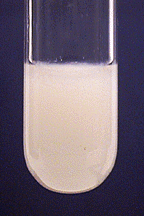# Confirmatory Tests

•• Contributed by James P. Birk
• Professor Emeritus (Chemistry) at Arizona State University

Confirmatory tests should be performed on separate solutions of some of your ions, in order to see what these tests look like before using them on an unknown. Generally a confirmatory test is used only after other reactions have been used to isolate the ion. When working with stock solutions of an ion, dilute 1 drop with 9 drops of water to simulate the concentration that would exist in an unknown. In a mixture or a solution obtained from an unknown or known mixture, the dilution is not necessary since the ions have already been diluted compared to the stock solutions. In the tests described here, it is assumed that 10 drops of solution will be used. If you change the amount of solution of the ion being tested, you must also adjust the amounts of the reagents to be added.

### A. Tests Based on Hydrogen Sulfide

Even if hydrogen sulfide was not used for separation of ions, it may be useful for confirmatory tests. The most convenient and safe source of $$\ce{H2S}$$ is thioacetamide. When heated, aqueous solutions of thioacetamide hydrolyze to produce $$\ce{H2S}$$:

In acid solution:

$\ce{CH3CSNH2 + 2H2O + H^{+} -> CH3COOH + NH4^{+} + H2S}$

In basic solution using ammonia:

$\ce{CH3CSNH2 + 2H2O + 2NH3 -> CH3COO^{-} + 3NH4^{+} + S2^{-}}$

In basic solution using strong base:

$\ce{CH3CSNH2 + 2OH^{-} -> CH3COO^{-} + NH3 + S2^{-} + H2O }$

### Antimony (Sb³⁺)

To 10 drops of solution, add 6 M $$\ce{NH3(aq)}$$ until neutral. Make the solution acidic by adding one or more drops of 6 M $$\ce{HCl}$$. Add 1 mL of thioacetamide and stir well. Heat the test tube in the boiling water bath for 5 minutes. If antimony is present, a red orange precipitate of antimony sulfide should form. This same test will also work with arsenic(III),

tin(II),and tin(IV).These precipitates are yellow, brown, and yellow, respectively.

Three related procedures can be used.

a. Follow the procedure described for antimony(III). The precipitate should be yellow.b. Follow the procedure described for antimony(III), but first make the solution basic with aqueous ammonia. If a precipitate forms on addition of ammonia, continue to add ammonia until the precipitate dissolves, before adding the thioacetamide.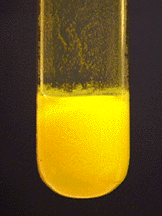c. Add ammonia, as described in procedure b. Then add 10 drops of water and 10 drops of 6 M $$\ce{NaOH}$$. A white precipitate should form. If it does not form, either increase the amount of $$\ce{NaOH}$$, or do not add the 10 drops of water. Centrifuge and discard the solution. Wash the precipitate twice with a mixture of 1 mL of water and 1 mL of 6 M $$\ce{NH3(aq)}$$. Dissolve the precipitate by adding 6 M $$\ce{HCl}$$ drop by drop until no precipitate remains. Add 6 M $$\ce{NaOH}$$ until the solution is just basic. A white precipitate of $$\cd{Cd(OH)2}$$ will form. Then add 1 mL of 1 M thioacetamide and heat the mixture in a boiling water bath for 5 minutes. A yellow precipitate of $$\ce{CdS}$$ will form.### Mercury(II) (Hg²⁺)

Follow the procedure described for antimony(III). The precipitate should be black.Try to dissolve the precipitate in 1 mL of 12 M $$\ce{HCl}$$ with heating. If it does not dissolve in $$\ce{HCl}$$, try the same procedure with 1 mL of 6 M (dilute) $$\ce{HNO3}$$. If it still does not dissolve, then try to dissolve it in a mixture of 1 mL of 6 M $$\ce{HCl}$$ and 1 mL of 6 M $$\ce{HNO3}$$, heating for 2 minutes in a water bath. Most of the black precipitate should dissolve. Mercury(II) sulfide is the least soluble of the metal sulfides.## Tests Based on Other Reagents

### Aluminum (Al³⁺)

To 10 drops of solution, add 2 drops of aluminon. Add 6 M $$\ce{NH3(aq)}$$ dropwise until the solution is basic to litmus. White $$\ce{Al(OH)3}$$ should form and adsorb the aluminon, which colors it red. The solution should become colorless.### Ammonium (NH₄⁺)

Place 10 drops of solution in a 30 mL beaker. Place a moistened piece of red litmus paper and put it on the bottom of a small watch glass. Add 1 mL of 6 M $$\ce{NaOH}$$ to the sample in the beaker. Cover the beaker with the watch glass. Gently heat the solution to near the boiling point. Do not allow the solution to splatter onto the litmus paper. The paper should turn blue from ammonia fumes.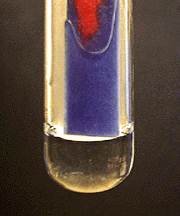### Bismuth (Bi³⁺)

Two procedures can be used.

a. To 10 drops of solution, add a freshly prepared sodium stannite solution dropwise. A black precipitate should form.Sodium Stannite: The sodium stannite solution is prepared by diluting 4 drops of 0.25 M $$\ce{SnCl2}\0 with 2 mL water and adding 6 M \(\ce{NaOH}$$ dropwise, stirring well after each drop, until a permanent precipitate forms. Then add excess NaOH to dissolve this precipitate.

b. To 10 drops of solution, add 2 drops of 6 M $$\ce{HCl}$$. Then add water dropwise until a white precipitate forms. The precipitate may not be very pronounced, but may instead show up as a turbidity of the solution.### Calcium (Ca²⁺)

Two procedures may be used.

a. To 10 drops of solution, add aqueous ammonia to make the solution basic. Then add $$\ce{(NH4)2C2O4}$$ (ammonium oxalate) solution dropwise. A white precipitate should form.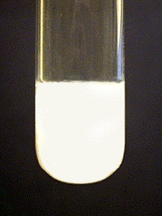b. Perform a flame test. The flame should turn orange-red.

### Chromium (Cr³⁺)

To 10 drops of solution, add 1 mL of 3% $$\ce{H2O2}$$. Then add 6 M $$\ce{NaOH}$$ dropwise until the solution is basic. Heat in a boiling water bath for a few minutes. A yellow solution of $$\ce{CrO4^{2-}}$$ should form.### Cobalt (Co²⁺)

To 10 drops of solution, add 5 drops of 0.5 M $$\ce{KNCS}$$. To this mixture, add an equal volume of acetone and mix. A blue color indicates the formation of $$\ce{[Co(NCS)4]^{2-}$$.### Copper (Cu²⁺)

To 10 drops of solution, add 0.5 M $$\ce{K4Fe(CN)6}$$ dropwise until a red-brown precipitate forms.### Iron (Fe³⁺)

Two procedures can be used.

a. To 10 drops of solution, add 0.5 M $$\ce{K4Fe(CN)6}$$ dropwise until a dark blue precipitate forms.b. To 10 drops of solution, add 1 or 2 drops of 0.5 M $$\ce{KNCS}$$. The solution should become deep red due to formation of $$\ce{FeNCS^{2+}}$$.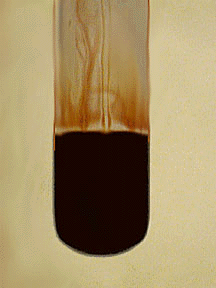### Magnesium (Mg²⁺)

To 10 drops of solution, add 2 drops of magnesium reagent, 4-(p-nitrophenylazo)resorcinol. Then add 6 M $$\ce{NaOH}$$ dropwise. A "blue lake" -- a precipitate of $$\ce{Mg(OH)2}$$ with adsorbed magnesium reagent -- forms.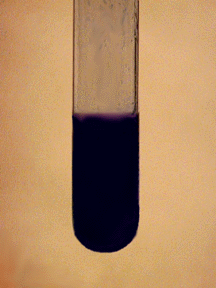### Manganese (Mn²⁺)

To 10 drops of solution, add 1 mL of 6 M $$\ce{HNO3}$$. Add a spatula-tip quantity of solid sodium bismuthate ($$\ce{NaBiO3}$$) and stir well. There should be a slight excess of solid bismuthate. Wait about 1 minute and then centrifuge the mixture. The solution should be purple due to the presence of $$\ce{MnO4^{-}}$$.### Mercury(I) (Hg₂²⁺)

To 10 drops of solution, add 6 M $$\ce{HCl}$$ to form a white precipitate. Centrifuge and discard the centrifugate. Add 6 M $$\ce{NH3(aq)}$$ to the precipitate. The color of the precipitate should change to gray or black due to formation of mercury metal.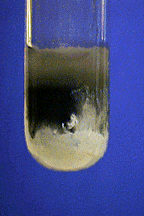### Mercury(II)(Hg²⁺)

To 10 drops of solution, add 1 or more drops of 0.25 M $$\ce{SnCl2}$$. A grayish precipitate should form. The precipitate might be white or black instead of gray.### Nickel (Ni²⁺)

To 10 drops of solution, add 6 M $$\ce{NH3(aq)}$$ until the solution is basic. Add 2 or 3 drops of dimethylglyoxime reagent (DMG). A rose-red precipitate of $$\ce{Ni(DMG)2}$$ should form.### Silver (Ag⁺)

To 10 drops of solution, add 6 M $$\ce{HCl}$$ dropwise, with shaking, until precipitation is complete. Centrifuge and decant. Discard the centrifugate. Suspend the silver chloride precipitate in 1 mL of water and add 6 M $$\ce{NH3(aq)}$$ dropwise until the precipitate dissolves. Acidify the solution with 6 M $$\ce{HNO3}$$ and the white precipitate should reappear.### Strontium (Sr²⁺)

To 10 drops of the solution , add 5 drops of ethanol ($$\ce{C2H5OH}$$). Then add 3 M (6 N) $$\ce{H2SO4}$$ dropwise until precipitation is complete. Heat the sample in a water bath for a few minutes. Test again for complete precipitation by adding 1 more drop of sulfuric acid. Centrifuge and decant the supernatant. If any further tests will be carried out on the supernatant liquid, heat it in a boiling water bath to expel the $$\ce{C2H5OH}$$, which could interfere with further tests. The white precipitate of $$\ce{SrSO4}$$ confirms the presence of strontium.### Tin (Sn²)

To 10 drops of solution, add 1 or 2 drops of 0.2 M $$\ce{HgCl2}$$. A white precipitate should form, but it could be gray or black instead.### Zinc (Zn²⁺)

To 10 drops of solution, add 6 M $$\ce{NH3(aq)}$$ to give a neutral pH. Then make the solution slightly acidic to litmus paper with 6 M $$\ce{HCl}$$. Add 1 or 2 drops of 0.5 M $$\ce{K4Fe(CN)6}$$ and stir. A gray-white precipitate of $$\ce{K2Zn[Fe(CN)6]2}$$ is formed.